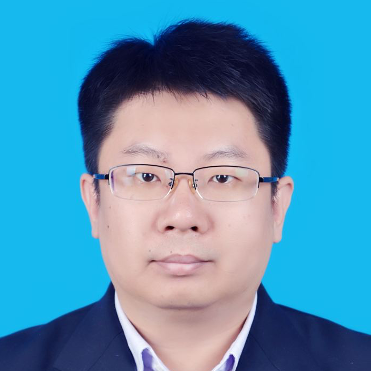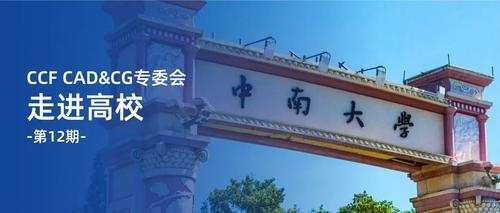## 雷娜、方玉明、张举勇、周元峰走进中南大学

### 特邀报告1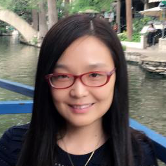In this talk we first introduce an optimal transportation (OT) view of deep learning. Natural datasets have intrinsic patterns, which can be summarized as the manifold distribution principle: the distribution of a class of data is close to a low-dimensional manifold. Deep learning mainly accomplish two tasks: manifold learning and probability distribution transformation. The latter can be carried out using the classical OT method. From the OT perspective, the generator of GAN model computes the OT map, while the discriminator computes the Wasserstein distance between the generated data distribution and the real data distribution; both can be reduced to a convex geometric optimization process. Furthermore, OT theory discovers the intrinsic collaborative—instead of competitive—relation between the generator and the discriminator.  Then we give an explanation to mode collapse. By the  regularity theory of Monge-Ampere equation the OT map is discontinued if the support of the target distribution is non-convex. But the neural network always represents continue map. This is the reason of mode collapse. Finally based on the above theory we propose three novel models, including generative model AE-OT, super resolutin model OTSR and point cloud upsampling model PU-CycGAN.

### 特邀报告2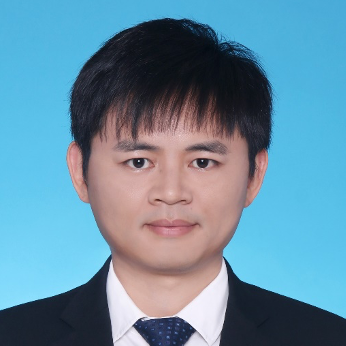### 特邀报告3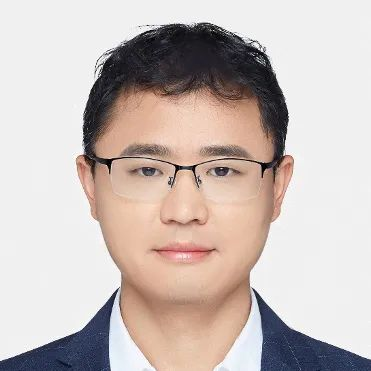### 特邀报告4